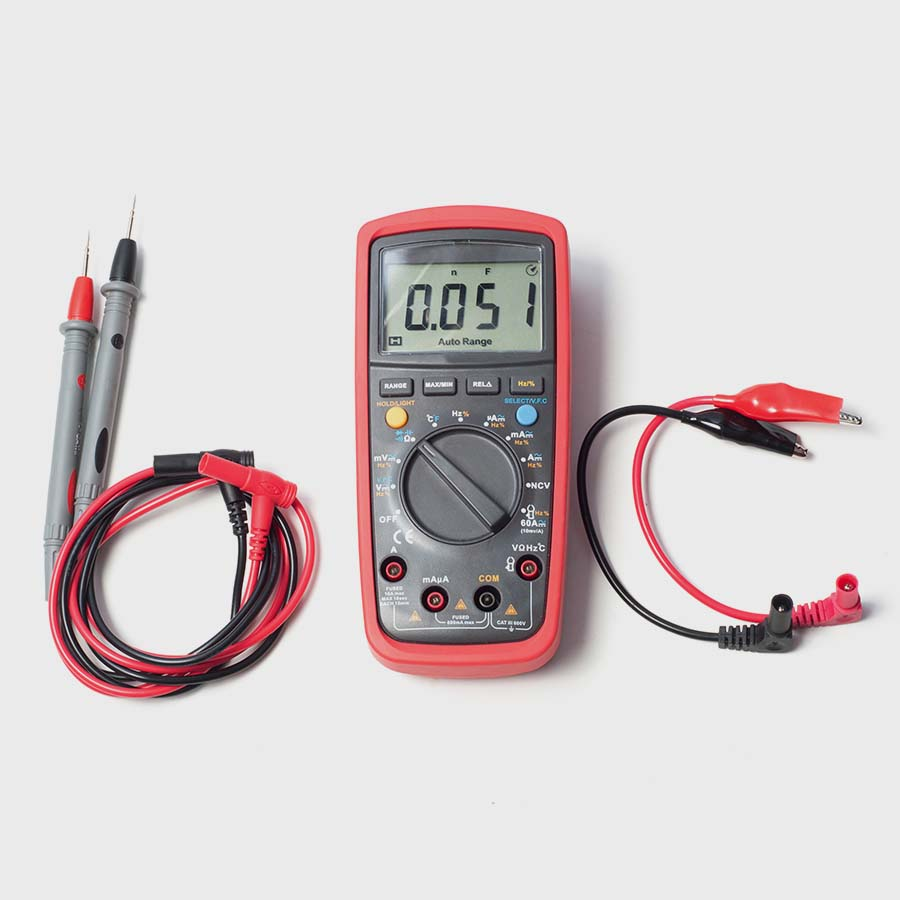\$30

Credit Hours: 2
Categories: , Valid in the following states: , ,

## Electricity 101- Electrical

Electricity 101 / Electronics 101 will give you an overview about basic principle of electronics. You will learn how electricity is produced, types of electricity, and important information about electrical circuits. You will learn the basic formulas that are used in the electronics field to figure the relationship between, power, voltage, resistance and current. Have you wondered how a transformer works or how to get more amp hours from a battery or even how a volt ohm meter works? By the end of the course you will have a basic understanding of all of these items with fundamental skills you can use in your every day life.

This course is to be applied to an Electrical License renewal only.

Categories: , Valid in the following states: , ,## Product Description / Course Objectives

• Learn how electricity is produced
• Understand the job of conductors and insulators
• Understand resistance and the impact of resistors in an electrical circuit
• Learn about Ohms law and Watts law
• Learn the basic formulas to figure the relationship between power, voltage, resistance and current
• Understand how a transformer works
• Comprehend the basics of batteries
• Understand simple circuits and switches we use every day
• Apply volt ohm meter basics.

This course is to be applied to an Electrical License renewal only.

#### Approved for CEU Credit in:

Delaware, South Dakota, Wyoming